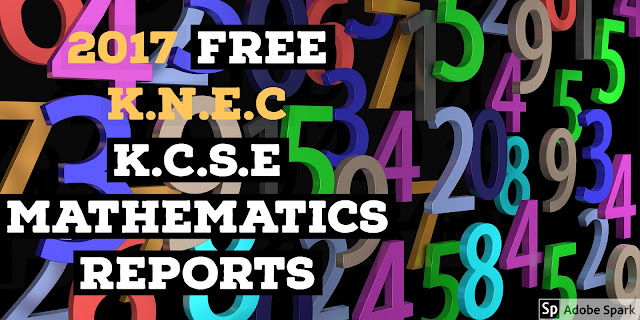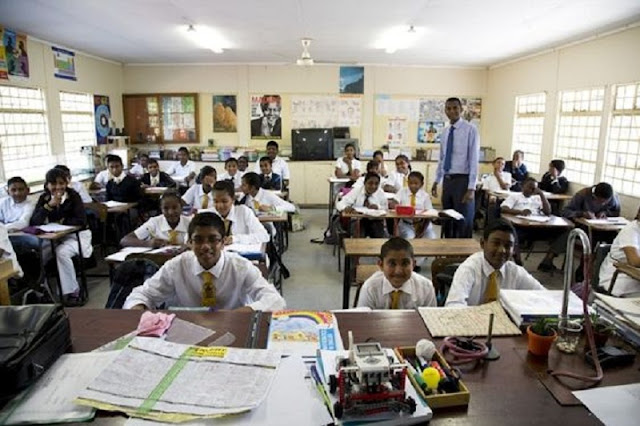## 2017 FREE K.N.E.C K.C.S.E MATHEMATICS REPORTS

### 2017 MATHEMATICS ALT A REPORTS FEATURED:ALT A
1. Factorization
2. Ratios, Percentage Profits and proportions
3. Linear Inequalities
5. Area approximations by mid ordinate rule
6. Calculations of errors
7. Locus
8. Statistics
9. Probability
10. Collinearity of Vectors
11. Transformations - Sheer and Stretch
12. Linear Programming
ALT B
1. Probability
2. Trigonometry (Sine and Cosine rule)
3. Matrix
4. Area of a Circle